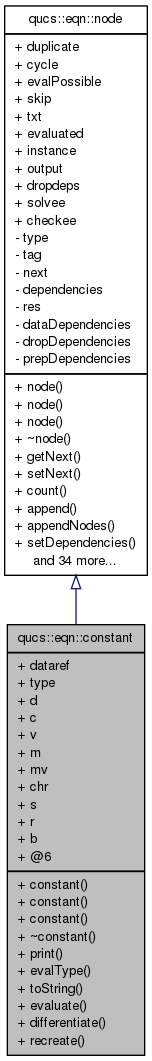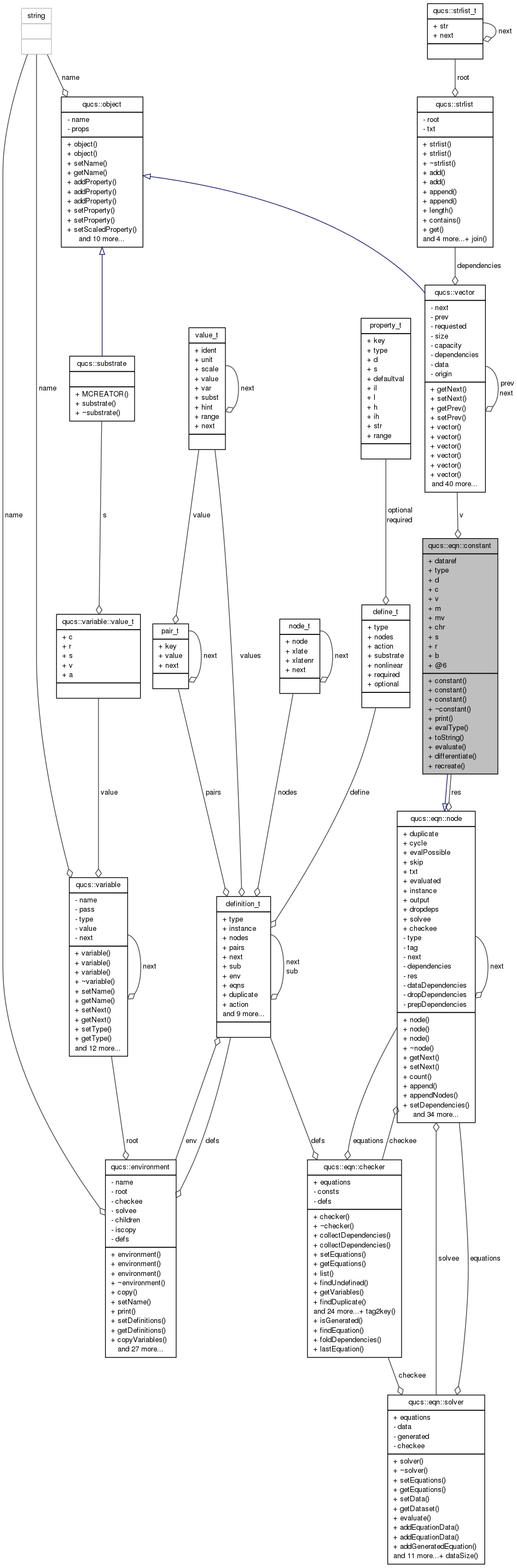Qucs-core  0.0.19
qucs::eqn::constant Class Reference

`#include <equation.h>`

Inheritance diagram for qucs::eqn::constant:[legend]
Collaboration diagram for qucs::eqn::constant:[legend]

Public Member Functions

constant ()
constant (int)
constant (const constant &)
~constant ()
void print (void)
int evalType (void)
char * toString (void)
constantevaluate (void)
nodedifferentiate (char *)
noderecreate (void)

Data Fields

bool dataref
int type
union {
nr_double_t   d
nr_complex_t *   c
qucs::vector *   v
matrix *   m
matvec *   mv
char   chr
char *   s
range *   r
bool   b
};

Detailed Description

Definition at line 151 of file equation.h.

Constructor & Destructor Documentation

 qucs::constant::constant ( )

Definition at line 63 of file equation.cpp.

 qucs::constant::constant ( int tag )

Definition at line 72 of file equation.cpp.

 qucs::constant::constant ( const constant & o )

Definition at line 82 of file equation.cpp.

 qucs::constant::~constant ( )

Definition at line 127 of file equation.cpp.

Member Function Documentation

 node * qucs::constant::differentiate ( char * ) ` [virtual]`

Reimplemented from qucs::eqn::node.

Definition at line 269 of file equation.cpp.

 int qucs::constant::evalType ( void ) ` [virtual]`

Reimplemented from qucs::eqn::node.

Definition at line 256 of file equation.cpp.

 constant * qucs::constant::evaluate ( void ) ` [virtual]`

Reimplemented from qucs::eqn::node.

Definition at line 262 of file equation.cpp.

 void qucs::constant::print ( void ) ` [virtual]`

Reimplemented from qucs::eqn::node.

Definition at line 157 of file equation.cpp.

 node * qucs::constant::recreate ( void ) ` [virtual]`

Reimplemented from qucs::eqn::node.

Definition at line 121 of file equation.cpp.

 char * qucs::constant::toString ( void ) ` [virtual]`

Reimplemented from qucs::eqn::node.

Definition at line 180 of file equation.cpp.

Field Documentation

 union { ... }

Definition at line 177 of file equation.h.

Definition at line 170 of file equation.h.

Definition at line 174 of file equation.h.

Definition at line 169 of file equation.h.

Definition at line 166 of file equation.h.

 matrix* qucs::eqn::constant::m

Definition at line 172 of file equation.h.

 matvec* qucs::eqn::constant::mv

Definition at line 173 of file equation.h.

Definition at line 176 of file equation.h.

 char* qucs::eqn::constant::s

Definition at line 175 of file equation.h.

Reimplemented from qucs::eqn::node.

Definition at line 167 of file equation.h.

 qucs::vector* qucs::eqn::constant::v

Definition at line 171 of file equation.h.

The documentation for this class was generated from the following files: